##### Pre-Calculus For Dummies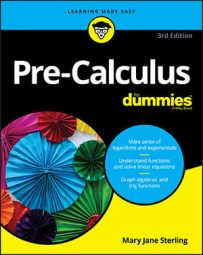If you have a coefficient tied to a variable on one side of a matrix equation, you can multiply by the coefficient's inverse to make that coefficient go away and leave you with just the variable. For example, if 3x = 12, how would you solve the equation? You'd divide both sides by 3, which is the same thing as multiplying by 1/3, to get x = 4. So it goes with matrices.

In variable form, an inverse function is written as f –1(x), where f –1 is the inverse of the function f. You name an inverse matrix similarly; the inverse of matrix A is A–1. If A, B, and C are matrices in the matrix equation AB = C, and you want to solve for B, how do you do that? Just multiply by the inverse of matrix A (if the inverse exists), which you write like this:

A–1[AB] = A–1C

So the simplified version is B = A–1C.

Now that you've simplified the basic equation, you need to calculate the inverse matrix in order to calculate the answer to the problem.

First off, you must establish that only square matrices have inverses — in other words, the number of rows must be equal to the number of columns. And even then, not every square matrix has an inverse. If the determinant of a matrix is not 0, then the matrix has an inverse.

## How to find the inverse of a matrix

When a matrix has an inverse, you have several ways to find it, depending how big the matrix is. If the matrix is a 2-x-2 matrix, then you can use a simple formula to find the inverse. However, for anything larger than 2 x 2, you should use a graphing calculator or computer program (many websites can find matrix inverses for you').

If you don't use a graphing calculator, you can augment your original, invertible matrix with the identity matrix and use elementary row operations to get the identity matrix where your original matrix once was. These calculations leave the inverse matrix where you had the identity originally. This process, however, is more difficult.

With that said, here's how you find an inverse of a 2-x-2 matrix:

If matrix A is the 2-x-2 matrix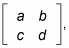its inverse is as follows: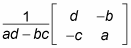Simply follow this format with any 2-x-2 matrix you're asked to find.

## How to solve the equations

Armed with a system of equations and the knowledge of how to use inverse matrices, you can follow a series of simple steps to arrive at a solution to the system, again using the trusty old matrix. For instance, you can solve the system that follows by using inverse matrices: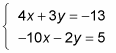These steps show you the way:

1. Write the system as a matrix equation.

When written as a matrix equation, you get2. Create the inverse of the coefficient matrix out of the matrix equation.

You can use this inverse formula:In this case, a = 4, b = 3, c = –10, and d = –2. Hence ad – bc = 22. Hence, the inverse matrix is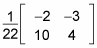3. Multiply the inverse of the coefficient matrix in the front on both sides of the equation.

You now have the following equation: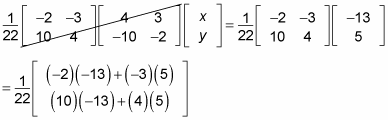4. Cancel the matrix on the left and multiply the matrices on the right.

An inverse matrix times a matrix cancels out. You're left with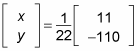5. Multiply the scalar to solve the system.

You finish with the x and y values: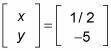Note that multiplying the scalar is usually easier after you multiply the two matrices.